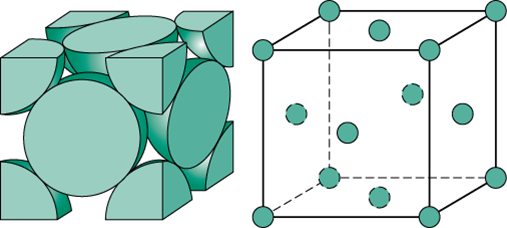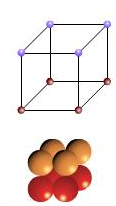#### An ionic compound has a unit cell consisting of A  ions at the corners of a cube and B ions on the centres of the faces of the cube. The empirical formula for this compound would be Option 1)Option 2)Option 3)Option 4)As we learnt in

No. of atoms(z) for face centered unit cell -

Lattice points: at corners and face centers of unit cell.

For face centered cubic (FCC), z=4.

- whereinNo. of atoms(z) for simple cubic unit cell -

Lattice points: at corners

For simple cubic (SC), z=1

- whereinNumber of A  ions per unit cellNumber of B ions per unit cellCorrect option is 3.

Option 1)This is an incorrect option.

Option 2)This is an incorrect option.

Option 3)This is the correct option.

Option 4)This is an incorrect option.

#### Sabhrant Ambastha